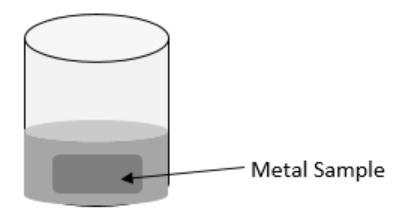# 23.4: Procedures

$$\newcommand{\vecs}{\overset { \rightharpoonup} {\mathbf{#1}} }$$ $$\newcommand{\vecd}{\overset{-\!-\!\rightharpoonup}{\vphantom{a}\smash {#1}}}$$$$\newcommand{\id}{\mathrm{id}}$$ $$\newcommand{\Span}{\mathrm{span}}$$ $$\newcommand{\kernel}{\mathrm{null}\,}$$ $$\newcommand{\range}{\mathrm{range}\,}$$ $$\newcommand{\RealPart}{\mathrm{Re}}$$ $$\newcommand{\ImaginaryPart}{\mathrm{Im}}$$ $$\newcommand{\Argument}{\mathrm{Arg}}$$ $$\newcommand{\norm}{\| #1 \|}$$ $$\newcommand{\inner}{\langle #1, #2 \rangle}$$ $$\newcommand{\Span}{\mathrm{span}}$$ $$\newcommand{\id}{\mathrm{id}}$$ $$\newcommand{\Span}{\mathrm{span}}$$ $$\newcommand{\kernel}{\mathrm{null}\,}$$ $$\newcommand{\range}{\mathrm{range}\,}$$ $$\newcommand{\RealPart}{\mathrm{Re}}$$ $$\newcommand{\ImaginaryPart}{\mathrm{Im}}$$ $$\newcommand{\Argument}{\mathrm{Arg}}$$ $$\newcommand{\norm}{\| #1 \|}$$ $$\newcommand{\inner}{\langle #1, #2 \rangle}$$ $$\newcommand{\Span}{\mathrm{span}}$$$$\newcommand{\AA}{\unicode[.8,0]{x212B}}$$

You will analyze the results of placing hot metal samples into cold water.

1. Draw a table in which to collect all of your data.  Do not fill in data until you have read the instructions for obtaining that data.

Table $$\PageIndex{1}$$: Temperature Data

Sample

Mass Metal Sample

(grams)

Mass Cold Water

(grams)

$$T_i$$ Water

(Celsius)

$$T_i$$ Metal

(Celsius)

$$T_f$$ Both

(Celsius)

$$\Delta T$$ Metal

$$\Delta T$$ Water

1. Choose three metal samples. List the material or color for each metal sample. Measure and record the mass in grams for each of your metal samples.

2. Place your first metal sample into the beaker and add water until your metal is completely submerged in water.  Place the beaker on the heat source, cover with a foil lid, and heat until boiling.  While you are waiting for the water to boil, read the procedures that follow so you are ready for the transfer.Figure $$\PageIndex{1}$$
1. Use the graduated cylinder to measure an amount of cold water that you think will completely cover your metal sample when the metal sample is placed into the Styrofoam cups.  Keep track of the amount of water you have in mL.  Add the cold water from the graduated cylinder to the Styrofoam cup, while your metal is heating in the beaker.  Record the total mL you add to the Styrofoam cup as the mass cold water in grams.  (1 mL = 1 gram of water)

2. Place one thermometer into the cold water, in the Styrofoam cup.  Measure the temperature in Celsius of the cold water in the Styrofoam cup, and record this value under $$T_i$$ Water.

Warnings

• Do not place a thermometer in a beaker while the beaker is on the heat source.
• Always assume your heat source is hot, even if it is off.
1. Once the water in the beaker has boiled for at least 2 minutes, to ensure that the metal sample is hot, follow the next steps as quickly as you can while being safe.

1. Remove the thermometer from the Styrofoam cup

2. Transfer the metal sample from the beaker  into the Styrofoam cup

3. Cover the Styrofoam cup with foil, seal the foil onto the Styrofoam cup with the rubber band, and carefully poke the thermometer through the foil making as small of a hole as possible (you are isolating your system of hot metal and cold water)

4. Remove the beaker with hot water from the heat source, and place the 2nd thermometer into the hot water

2. Observe both thermometers, the one in the Styrofoam cup and the one in the beaker.  When it looks like the temperatures have settled, record the temperatures in the appropriate places, in your data table.

• The temperature of the hot water is the assumed initial temperature for the hot metal sample, $$T_i$$Metal.

• The temperature in the Styrofoam cup with the water and metal is the final temperature for both the water and the metal, $$T_f$$ Both.

3. Repeat the processes to obtain data for your other metal samples.  You may re-use the hot water in the beaker to heat your second metal sample; make sure there is enough water to completely cover your next sample.  You will need to obtain new cold water in the Styrofoam cup for each sample.

4. Calculate and record the change in temperature ($$\Delta T$$ Water) for the cold water that occurred with each metal sample.  This will be the difference between $$T_f$$ Both and $$T_i$$Water.

5. Calculate and record the change in temperature ($$\Delta T$$ Metal) for each metal sample.  This will be the difference between $$T_f$$ Both and $$T_i$$ Metal.

## Clean-up

• Discard water outside (water plants)
• Wash and dry metal samples and beaker
• Wash and dry thermometers before replacing them in protective containers
• Completely dry Styrofoam cup
• Throw away aluminum foil lids